# [JustForFunPython] Classifying languages based on the frequency of each alphabet letter

`from sklearn imoprt svm, metrics import glob, os.path, re, json`
`def check_freq(fname):    name = os.path.basename(fname)     lang = re.match(r'^[a-z]{2,}', name).group()     with open(fname, 'r', encoding = 'utf-8') as f:        text = f.read()     text = text.lower()`
`def check_freq(fname):    name = os.path.basename(fname)     lang = re.match(r'^[a-z]{2,}', name).group()     with open(fname, 'r', encoding = 'utf-8') as f:        text = f.read()     text = text.lower()    cnt = [0 for n in range(0, 26)]     code_a = ord('a')    code_b = ord('z')    for ch in text:         n = ord(ch)         if code_a <= n <= code_z:            cnt[n-code_a] += 1`
`def check_freq(fname):    name = os.path.basename(fname)     lang = re.match(r'^[a-z]{2,}', name).group()     with open(fname, 'r', encoding = 'utf-8') as f:        text = f.read()     text = text.lower()    cnt = [0 for n in range(0, 26)]     code_a = ord('a')    code_b = ord('z')    for ch in text:         n = ord(ch)         if code_a <= n <= code_z:            cnt[n-code_a] += 1    total = sum(cnt)    freq = list(map(lambda n: n/total, cnt))     return (freq, lang)`
`def load_files(path):    freqs = []    labels = []     file_list = glob.glob(path)     for fname in file_list:         r = check_freq(fname)         freqs.append(r)         labels.append(r)    return {"freqs": freqs, "labels": labels}`
`data = load_files("./train/*.txt")test = load_files("./test/*.txt")with open("freq.json", "w", encoding = 'utf-8') as f:    json.dump([data, test], f)`
`clf = svm.SVC(gamma = 'auto') clf.fit(data['freq'], data['labels'])`
`predict = clf.predict(test['freqs'])`
`accuracy_score = metrics.accuracy_score(test['labels'], predict) classification_report = metrics.classification_report(test['labels'], predict)`

--

--

--

## More from A Ydobon

Ydobon is nobody.

Love podcasts or audiobooks? Learn on the go with our new app.

## Intro to ML Part 3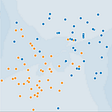## Modelling tabular data with Google’s TabNet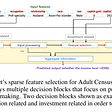## DL01: Neural Networks Theory## Training thousands of forecasting models at scale using Azure Automated ML and ParallelRunStep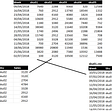## Optimization Algorithms## Experience on Implementing a state of art Deep Learning model using TensorFlow 2.0## Triggering Events with Facial Recognition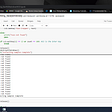## A Ydobon

Ydobon is nobody.

## The purpose of Activation functions in neural networks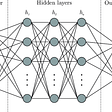## ML-Backpropagation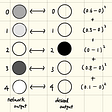## K-Nearest Neighbor in Machine Learning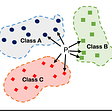## Let’s Learn: Neural Nets #3 — Activation Functions